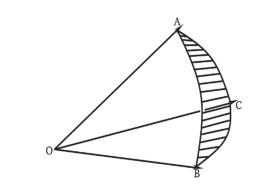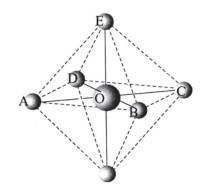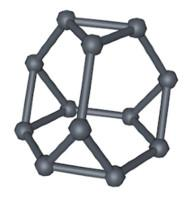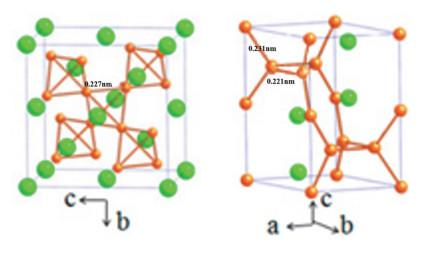## Application of Solid Angle in Structural ChemistryAbstract

The concept of solid angle is used to explain the necessary and sufficient conditions for a three-dimensional gapless structure to be achieved by combining one or several polyhedrons. In this paper, the solid angle and dihedral angle of the common regular polyhedron and the common Archimedean semi regular polyhedron are presented, and the examples of structural chemistry are analyzed. In space, the sum of solid angles must be equal to 4π(sr), so by simply adding, we can judge the possibility of gapless accumulation, know the common situation of each point, and then deduce the space structure. By using this method, we can predict some structures that do not exist yet.

Keywords： Solid angle ; Polyhedral ; Structural chemistry

Ma Zhongjie. Application of Solid Angle in Structural Chemistry. University Chemistry[J], 2020, 35(9): 209-213 doi:10.3866/PKU.DXHX201910004

## 1 立体角定义与计算公式

${\mathit{Ω}}_{\text{max}}\text{}\text{=}\text{}\text{4}\text{π}R^{\text{2}}\text{/}R^{\text{2}}\text{}\text{=}\text{}\text{4π(}sr\text{)}$

$\text{Σ}{\mathit{Ω}}_{\text{i}}\text{}\text{=}\text{}\text{4π(}sr\text{)}$

$\text{tan}\text{(}\mathit{Ω}\text{/4}\text{)}\text{=}\sqrt{\text{tan(}s\text{/2)tan[(}s\text{}\text{-}\text{}\text{α}\text{)/2]tan[(}s\text{}\text{-}\text{}\text{β}\text{)/2]tan[(}s\text{}\text{-}\text{}\text{γ}\text{)/2]}}$

Ω为O点观察球面三角形ABC的立体角，α =∠BOC，β =∠AOC，γ =∠AOB，$s\text{}\text{=}\text{}\text{(}\text{α}\text{}\text{+}\text{}\text{β}\text{}\text{+}\text{}\text{γ}\text{)/2}$

### 图1## 2 常见多面体的立体角

### 图2多面体 表面组成 立体角 正四面体 34 0.1754π (记为θ) 正八面体 38 $\frac{2}{3}$π −$\frac{4}{3}$θ 立方体 46 $\frac{1}{2}$π 切角四面体 3464 $\frac{2}{3}$π −$\frac{1}{3}$θ 立方八面体 3846 $\frac{2}{3}$π +$\frac{2}{3}$θ 切角立方体 3886 $\frac{5}{6}$π +$\frac{1}{3}$θ 切角八面体 4668 1π

### 3.1 简单立方结构

$\text{8·1/2π}\text{}\text{=}\text{}\text{4π(}sr\text{)}$

### 图3### 图4### 图5### 图6### 3.4 Laves相

$\text{2}\text{θ}\text{}\text{+}\text{}\text{6·(2/3π - 1/3}\text{θ}\text{)}\text{}\text{=}\text{}\text{4π(}sr\text{)}$

### 图7### 图8## 4 充分性与必要性

(1)每个面被2个多面体共用，即不同多面体存在相同的面；

(2)每条棱与周围多面体的面形成的二面角之和等于2π；

(3)每个点周围的立体角之和等于4π(sr)。

ccp密堆积中的多面体由正四面体与正八面体组成，均存在正三角形面，符合条件(1)。用立体角加和等于4π(sr)计算出每个点被8个正四面体和6个正八面体共用，符合条件(3)。多面体与点数在空间中的数量比等于多面体在每个点周围的数量除以多面体的顶点数所得的比值，即1 : (8/4) : (6/6) =1 : 2 : 1 (点数：正四面体数:正八面体数= 1 : 2 : 1)。ccp最密堆积中每一个点的配位数等于12，意即每一个点对应着12/2 = 6条棱边。而每个点对应2个正四面体和一个正八面体，对应着一共2 × 6 + 12 = 24条棱边。因此计算出每条棱边平均被4个多面体共用。正四面体的二面角为72.53°，正八面体的二面角为109.47°，恰好每条棱边被2个八面体和2个正四面体共用。另一方面，多面体与点数在空间中的数量比也等于多面体在每条棱边周围的数量除以多面体的棱数所得的比值，计算结果(2/12) : (2/6) = 1 : 2与前文结果相符，再次验证条件(3)。这时我们可以肯定，正四面体与正八面体按2 : 1组合能够形成配位数为12的三维无隙结构。

 多面体 二面角1 二面角2 正四面体 72.53° – 正八面体 109.47° – 立方体 90° – 切角四面体 72.53° 109.47° 立方八面体 90° 54.74° 切角立方体 90° 54.74° 切角八面体 109.47° 125.26°

## 5 在预测结构上的应用

### 图9### 图10## 参考文献 原文顺序 文献年度倒序 文中引用次数倒序 被引期刊影响因子

Weisstein, E. W. L'Huilier's Theorem. (2020-02-07)[2020-2-21]. http://mathworld.wolfram.com/LHuiliersTheorem.html.

Gao G. Y. ; Ashcroft N. W. ; Miao M. S. ; Hoffmann R. J. Phys. Chem. C 2014, 118 (43), 25170.

/

 〈〉